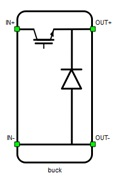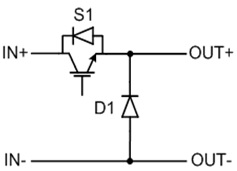# Buck

This section describes buck converters

A block diagram and input parameters for a buck converter block are given in Table 1>.

Table 1. Buck converter component in the Schematic Editor core library
component component dialog window component parametersBuck converter• Control (Digital inputs, Internal modulator, Model)
• S1 (1..32)
• S1_logic (active high, active low)
• Gate control enabling (enables usage of external digital signal to enable switching)
• Sen (digital input selection for gate enable)
• Sen_logic (active high, active low)
Weight = 1

A schematic block diagram of the buck switching block is given in Figure 1 with corresponding switch arrangement and naming.

Digital inputs, when selected for Control parameter, enables the user to assign gate drive inputs to any of the digital input pins (from 1 to 32). For example, if S1 is assigned to 1, the digital input pin 1 will be routed to the S1 switch gate drive. In addition, the S1_logic parameter selects either active high(High-level input voltage VIHturns on the switch) or active low (Low-level input voltage VILturns on the switch) gate drive logic, depending on the user’s external controller design.

Internal modulator, when selected for Control parameter, enables the user to use internal PWM modulator for driving S1 switch instead of digital input pins. In this configuration, two additional component inputs will be present. En input is used as enable/disable and In is used as referent signal input for internal PWM modulator.

Model, when selected for Control parameter, enables the user to set the IGBT gate drive signal directly from signal processing model. The input pin gate appears on the component. It is one element input, where that value controls S1 switch gate. When controlled from the model, logic is always active high.Figure 1. Schematic block diagram of a buck switching block with corresponding switch naming

## Losses calculation

When Losses calculation property is enabled, component will calculate switching and conduction power losses for all switching elements(igbts and diodes). Switching power losses are calculated as function of current, voltage and temperature using 3D Look-up tables. Also 2D input for losses is supported. When 2D losses table is inserted it assumes only current and temperature dependance. Conduction power losses are calculated based on Vce and Rce. Import options and explanation how to correctly fill all necessary power losses parameters is described in import power losses section. Input/output ports for power losses are vectors of two elements. First element (index 0) is IGBT and the second element (index 1) is diode. Availabe mask properties are:
• Vce - IGBT collector emitter saturation voltage [V]
• Rce - IGBT on state slope resistance [Ohm]
• Vd - Diode voltage drop [V]
• Rd - Diode slope resistance [Ohm]
• Current values - IGBT switching losses, current axis [A]
• Voltage values - IGBT switching losses, voltage axis [V]
• Temp values - IGBT switching losses, temperature axis [°C]
• Et on table out - IGBT switching ON losses, output energy, f(I, V, T) [J]
• Et off table out - IGBT switching OFF losses, output energy, f(I, V, T) [J]
• Ed off table out - Diode switching OFF losses, output energy, f(I, V, T) [J]

## Temperatures calculation

When Temperatures calculation property is enabled, component will calculate combined power losses (P_loss) and junction temperatures (T_junctions) for all switching elements (IGBTs and diodes). Combined power losses represent sum of the calculated switching and conduction losses transfered through internally generated Thermal network component. Internally generated Thermal network component also calculates junction temperatures from power losses, input cases temperatures and provided thermal model parameters. Input/output ports for temperatures calculation are vectors of 2 elements and they are indexed in the same way as explaind in the Losses calculation section. Additional temperatures calculation mask properties are:
• Thermal networks type - Defines type of internal thermal network
• Rth switch - List of thermal resistance for the IGBT switch
• Tth switch / Cth switch - List of thermal time constants or thermal capacitances for the IGBT switch
• Rth diode - List of thermal resistance for diode
• Tth diode / Cth diode - List of thermal time constants or thermal capacitances for diode
• Caculations execution rate - Execution rate in [s] for the losses and temperatures calculation logic

## Digital Alias

If a converter is controlled by digital inputs, an alias for every digital input used by the converter will be created. Digital input aliases will be available under the Digital inputs list alongside existing Digital input signals. The alias will be shown as Converter_name.Switch_name, where Converter_name is name of the converter component and Switch_name is name of the controllable switch in the converter.